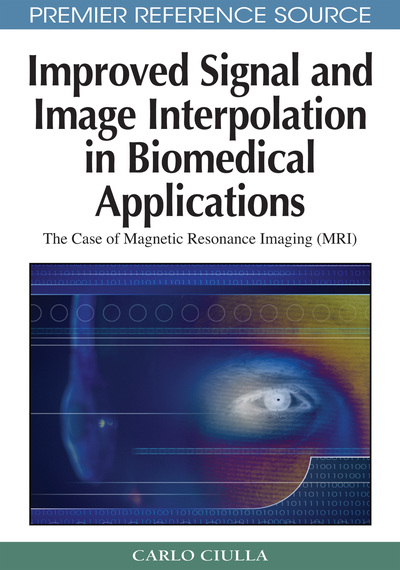# The Extension of Theory and Methodology to B-Splines

Carlo Ciulla (Lane College, USA)
DOI: 10.4018/978-1-60566-202-2.ch014

## Abstract

The organization of the chapter is similar to that of Chapters VII and X. The methodological approach to extend the unifying theory to the one dimensional quadratic and cubic B-Splines is herein reported along with the most relevant mathematical details. This chapter should be read along with Appendix VI where proofs are given to the assertions herein presented. In either of the two cases: quadratic and cubic B-Spline the math process starts from the calculation of the Intensity-Curvature Functional and continues with the calculation of the Sub-pixel Efficacy Region. Finally, the math process arrives to the calculation of the novel re-sampling locations through the formulas of the unifying theory seen in equations (23) and (33) for the quadratic and the cubic models respectively. The chapter concludes with a section that addresses specifically the theoretical proposition of resilient interpolation for the two classes of B-Splines. This is conducted consistently with Chapters VII and XII of the book choosing to equate the two intensity-curvature terms (before and after interpolation) as the starting point of the math deduction.
Chapter Preview
Top